In :
import pandas as pd
import numpy as np
import matplotlib
import matplotlib.pyplot as plt

from sklearn import tree

%matplotlib inline


## 9.1 Exponential loss vs misclasification loss¶

In boosting, we label (for convenience) the classes as $+1$ and $-1$, respectively. We furthermore let our classifier $\widehat{y}(x)$ be on the form $\widehat{y}(x) = \operatorname{sign}\left(c(x)\right)$, i.e., a thresholding at $0$ of some real-valued function $c(x)$. Assume that we have learned some function $\widehat{c}(x)$ from training data such that we can make predictions $\widehat{y}(x) = \operatorname*{sign}\left(\widehat{c}(x)\right)$. Fill in the missing columns in the table below:

${\widehat{c}{(x_\star)}}$ $\widehat{y}_\star$ Exponential loss
$\mathrm{exp}{\left(-y_\star\widehat{c}{(x_\star)}\right)}$
Misclassification loss
$\mathbb{I}\left(y_\star\neq\widehat{y}_\star\right)$
$y_\star$
0.3 -1
-0.2 -1
1.5 1
-4.3 1

In what sense is the exponential loss more informative than the misclassification loss, and how can that information be used when training a classifier?

### Solution¶

$\widehat{c}{(x_{\star})}$ $\widehat{y}_\star$ Exponential loss
$\mathrm{exp}{\left(-y_\star\widehat{c}{(x_\star)}\right)}$
Misclassification loss
$\mathbb{I}\left(y_\star\neq\hat{y}_\star\right)$
$y_\star$
0.3 1 exp(0.3)=1.35 1 -1
-0.2 -1 exp(-0.2)=0.82 0 -1
1.5 1 exp(-1.5)=0.22 0 1
-4.3 -1 exp(4.3)=73.70 1 1

Whereas the misclassification loss only gives the information correct or misclassified, the exponential loss contains the same information ($<1$: correct, $>1$ misclassfied) as well as information about the margin $y_\star c(x_\star)$, which in a sense gives an idea of "how much" wrong or correct the classifier is.

## 9.2 AdaBoost for spam classification¶

Consider the very same setting data set for the data set email.csv as in problem 8.2, but now use AdaBoost instead. AdaBoost is available in sklearn.ensemble as the command AdaBoostClassifier(). What test error do you achieve?

In :
# url = 'data/email.csv'
url = 'https://uu-sml.github.io/course-sml-public/data/email.csv'

In :
np.random.seed(1)
trainIndex = np.random.choice(email.shape, size=int(len(email)*.75), replace=False)
train = email.iloc[trainIndex]                     # training set
test = email.iloc[~email.index.isin(trainIndex)]      # test set

X_train = train.drop(columns=['Class'])
y_train = train['Class']
X_test = test.drop(columns=['Class'])
y_test = test['Class']

model.fit(X=X_train, y=y_train)
y_predict = model.predict(X_test)
print('Test error rate is %.3f' % np.mean(y_predict != y_test))

Test error rate is 0.054


## 9.3 Exploring the AdaBoost algorithm¶

The script below illustrates the AdaBoost algorithm in the same way as was done in lecture 7. Familiarize yourself with the code and compare it to the pseudocode given in the course literature (Chapter 7 in the lecture notes). Explore what happens if you make changes in the input data and the number of trees (stumps), B, used!

In :
X1 = np.array([0.2358,0.1252,0.4278,0.6398,0.6767,0.8733,0.3648,0.6336,0.3433,0.8410])
X2 = np.array([0.1761,0.4465,0.7539,0.9037,0.7111,0.8414,0.6060,0.5107,0.1430,0.1994])
X = np.column_stack([X1,X2])
y = np.array([1,1,1,1,1,-1,-1,-1,-1,-1])
n = len(y)
# note: the class labels are 1 and -1

fig, ax = plt.subplots()
ax.set_xlim((0, 1))
ax.set_ylim((0, 1))

# plot the data
ax.plot(X1[y==1], X2[y==1], 'x', color='red')
ax.plot(X1[y==-1], X2[y==-1], 'o', color='blue')

ax.set_xlabel('$X_1$')
ax.set_ylabel('$X_2$')

# define some variables we will fill out in the loop below
stumps = []
alpha = []
w = np.repeat(1/n, n) # the boosting weights, start with equal weights
B = 3

# learn the boosting classifier
for b in range(B):
# prepare plots
fig, ax = plt.subplots()
ax.set_xlim((0, 1))
ax.set_ylim((0, 1))
# use a decision stump (tree with depth 1) as base classifier
model = tree.DecisionTreeClassifier(max_depth=1)
model.fit(X, y, sample_weight=w)
stumps.append(model)                 # Saving the model in each step
# plot the decision boundary
split_variable = model.tree_.feature
split_value = model.tree_.threshold
if split_variable == 0:
x1 = [0, split_value, split_value, 0]
x2 = [0, 0, 1, 1]
ax.fill(x1, x2, 'lightsalmon', alpha=0.5)
x1 = [split_value, 1, 1, split_value]
ax.fill(x1, x2, 'skyblue', alpha=0.5)
elif split_variable == 1:
x1 = [0, 1, 1, 0]
x2 = [split_value, split_value, 1, 1]
ax.fill(x1, x2, 'lightsalmon', alpha=0.5)
x2 = [0, 0, split_value, split_value]
ax.fill(x1, x2, 'skyblue', alpha=0.5)

ax.set_xlabel('$x_1$')
ax.set_ylabel('$x_2$')

# plot the data
ax.scatter(X1[y==1], X2[y==1], s=50, facecolors='red')
ax.scatter(X1[y==-1], X2[y==-1], s=50, facecolors='blue')

# compute and plot the boosting weights for the next iteration
correct = model.predict(X) == y
ax.scatter(X1[~correct], X2[~correct], s=300, facecolors='none', edgecolors='k')

W = np.sum(w)
We = np.sum(w[~correct])   # Sum of incorrect weights

em = We/W
a = 1/2*np.log((1-em)/em)    # compute the alpha weights
alpha.append(a)

w[correct] = w[correct] * np.exp(-a)   # Omega weights
w[~correct] = w[~correct] * np.exp(a)

# Using the boosting model to predict test inputs
def boost_predict(X):
pred = []
for j in range(len(X)):
predictions = np.empty(B)
for i in range(B):
predictions[i] = stumps[i].predict(X[j,:].reshape(1,-1))
C = np.sum(np.array(alpha)[predictions==1]) - np.sum(np.array(alpha)[predictions==-1])
pred.append(np.sign(C))
return pred

# predict all pixels in the plot, i.e., plot the decision boundary
fig, ax = plt.subplots()
ax.set_xlim((0, 1))
ax.set_ylim((0, 1))
ax.set_xlabel('$x_1$')
ax.set_ylabel('$x_2$')
res = 0.1   # resolution of the squares
xs1 = np.arange(0, 1.02, 0.03)
xs2 = np.arange(0, 1.02, 0.03)
x1, x2 = np.meshgrid(xs1, xs2)
X_all = np.column_stack([x1.flatten(),x2.flatten()])
prediction = boost_predict(X_all)

colors = np.where(np.array(prediction)==1,'lightsalmon', 'skyblue')
ax.scatter(x1.flatten(), x2.flatten(), s = 90, marker='s', c=colors)
ax.scatter(X1[y==1], X2[y==1], s=50, facecolors='red')
ax.scatter(X1[y==-1], X2[y==-1], s=50, facecolors='blue')

plt.show()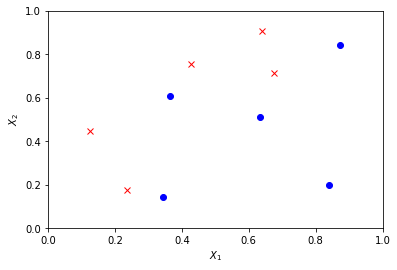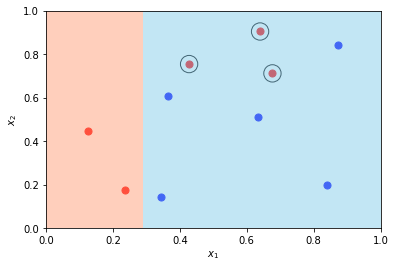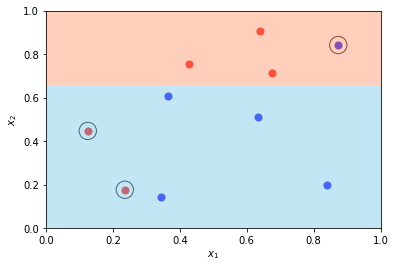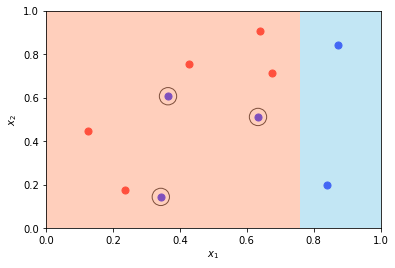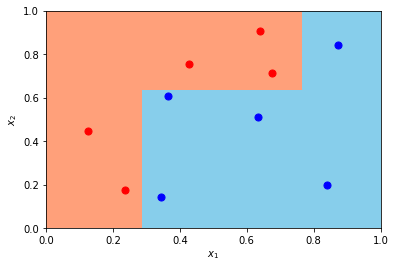## 9.4 Deriving the AdaBoost weights¶

The AdaBoost classifier can be written as \begin{equation} \widehat{y}_{\text{boost}}(\mathbf{x}) = \text{sign}\left( c^{(B)}(\mathbf{x}) \right) \end{equation} where the functions $c^{(1)}(\mathbf{x}), \, \dots, c^{(B)}(\mathbf{x})$ are constructed sequentially as \begin{equation} c^{(b)}(\mathbf{x}) = c^{(b-1)}(\mathbf{x}) + \alpha^{(b)} \widehat y^{(b)}(\mathbf{x}), \end{equation} initialized with $c^{(0)}(x) \equiv 0$. The $b$th ensemble member $\widehat y^{(b)}(x)$ is found by applying the chosen base classifier to a weighted version of the training data. Once this has been found, we also need to compute the corresponding "confidence" coefficient $\alpha^{(b)}$. This is done by minimizing the weighted exponential loss of the training data, \begin{align} \alpha^{(b)} = \arg\min_{\alpha}\left\{\sum_{i=1}^n w_i^{(b)} \exp \left(- \alpha y_i \widehat y^{(b)}(\mathbf{x}_i) \right) \right\} \end{align} where $w_i^{(b)} = \exp\left(-y_i c^{(b-1)}(\mathbf{x}_i)\right)$.

Show that the optimal solution is given by \begin{equation} \alpha^{(b)} = \frac{1}{2}\ln\left( \frac{1 - E_{\text{train}}^{(b)}}{E_{\text{train}}^{(b)}} \right) \end{equation} where \begin{equation} E_{\text{train}}^{(b)} = \sum_{i=1}^n \frac{w_i^{(b)}}{\sum_{j=1}^n w_{j}^{(b)}} \mathbb{I}(y_i \neq \widehat{y}^{(b)}(\mathbf{x}_i)). \end{equation}

_Hint 1: We use class labels $-1$ and $1$, i.e. $y_i \in \{-1, 1\}$ and $\widehat{y}^{(b)}(\mathbf{x}_i) \in \{-1, 1\}$. Using this fact, divide the sum in the objective function in the equation for $\alpha^{(b)}$ into one sum ranging over all correctly classified training data points and one sum ranging over all misclassified training data points._

Hint 2: You are allowed to use the fact that the objective function in the equation for $\alpha^{(b)}$ has a single stationary point corresponding to the global minima.

### Solution¶

We want to minimize the expression \begin{equation} F(\alpha) := \sum_{i=1}^n w_i^{(b)} \exp\left(- \alpha y_i \widehat{y}^{(b)}(\mathbf{x}_i)\right) \end{equation} with respect to $\alpha$. Using the second hint of the problem formulation, we can do this by differentiating the expression, setting the derivative to zero, and solving for $\alpha$.

Before we do this, however, we make use of the first hint of the problem formulation to simplify the expression under study. Note first that using the class labels $-1$ and $1$ implies that \begin{equation} y_i \widehat{y}^{(b)}(\mathbf{x}_i)= \begin{cases} 1 & \text{if } \widehat{y}^{(b)}(\mathbf{x}_i) = y_i,\\ -1 & \text{if } \widehat{y}^{(b)}(\mathbf{x}_i) \neq y_i. \end{cases} \end{equation} Thus, the expression for $F(\alpha)$ can be written as \begin{equation} F(\alpha) = e^{-\alpha} \underbrace{\sum_{i = 1}^n w_i^{(b)} \mathbb{I}\left( \widehat{y}^{(b)}(\mathbf{x}_i) = y_i\right)}_{=: W_c} + e^{\alpha} \underbrace{\sum_{i =1}^n w_i^{(b)} \mathbb{I}\left( \widehat{y}^{(b)}(\mathbf{x}_i) \neq y_i \right)}_{=: W_e}, \end{equation} where we have used the indicator function to split the sum into two sums: the first ranging over all correctly classified data points, and the second ranging over all erroneously classified data points. Furthermore, for notational simplicity we define $W_c$ and $W_e$ for the sum of weights of correctly classified data points and erroneously classified data points, respectively.

Now, we compute the derivative and set to zero: $$\frac{d F}{d\alpha} = -e^{-\alpha} W_c + e^\alpha W_e = 0 \Longleftrightarrow e^{\alpha} W_e = e^{-\alpha} W_c \Longleftrightarrow e^{2\alpha} = \frac{W_c}{W_e} \Longleftrightarrow \alpha = \frac{1}{2} \ln \left( \frac{W_c}{W_e} \right).$$ It remains to write the solution on the format asked for in the problem formulation. With $W := \sum_{i=1}^n w_i^{(b)}$ denoting the sum of all weights, we note first that $W_c = W - W_e$ and thus, \begin{align*} \alpha = \frac{1}{2} \ln \left( \frac{W - W_e}{W_e} \right) = \frac{1}{2} \ln \left( \frac{1 - W_e/W}{W_e/W} \right). \end{align*} Finally, noting that $E_{\text{train}}^{(b)} = W_e/W$ completes the proof.

Explore gradient boosting by using GradientBoostingClassifier() on the spam data set email.csv
model = GradientBoostingClassifier()

Test error rate is 0.052